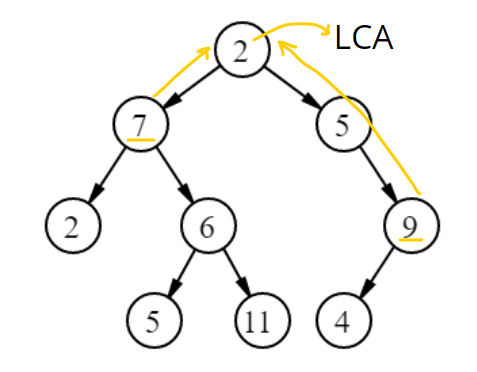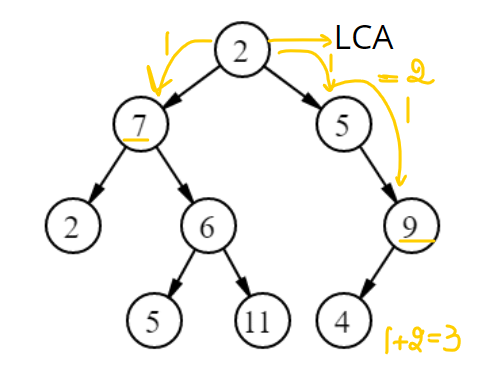# Find distance between two nodes of a Binary Tree

## Introduction-

Today, let's learn about a famous and commonly asked Interview Problem, i.e., find the distance between two nodes of a Binary Tree.

The binary tree is a generic tree with only two children, i.e., left and right.

Problem Statement: Given the root of the binary tree and the two nodes, we need to return the shortest distance between the two nodes.

Example:Let's consider the two given nodes as 7 and 9. The shortest distance between two nodes is three as-

7 -> 2 -> 5 -> 9.

Hence, we would reach 9 from 7 (or vice versa) in 3 steps.

The approach to solving this problem is straightforward, i.e., via first finding LCA(Least Common Ancestor) of the two given nodes and then calculating - (the distance between LCA and node1) + (the distance between LCA and node2).

This would be the shortest distance between two nodes of a Binary Tree.

## Approach

STEP 1: Calculate LCA(Least Common Ancestor)The lowest common ancestor(LCA) between two nodes n1 and n2 is defined as the lowest node in Binary Tree with both n1 and n2 as descendants (where we allow a node to be a descendant of itself).

To calculate the LCA of nodes 7 and 9, we first call a recursive function passing (the root of Binary Tree, Node1 i.e.7, Node2, i.e., 9).

• We first check in our base case if (root == null), stating we have a null tree or have reached a null node. If this is the case, we return null.
• Otherwise, we check if the root (or current node) equals Node1 or Node2. If it is so, we return the root.
• Otherwise, we further explore in both the left and the right direction. If both the directions return some non-null node, this means our LCA would be our current node(i.e., root). So we return the root node.
• Otherwise, we return the not null node we received from either of the sides or a null node if we received a null node from both the left and right subtrees denoting that they do not contain any of the nodes n1 and n2.

STEP 2: Find the distance of Node 1 and Node2 from the LCA.So, we again traverse the tree until we find the level from our LCA node, where our "to-found node" is located.

Finally, we sum both the distances (distance from LCA to Node1 and LCA to Node2) and return our answer(the shortest distance between two nodes of a Binary Tree).

Implementation-

Let’s have a look at its implementation in Java -

Output:

## Time and Space Complexity-

Time Complexity: O(n) where n is the number of nodes in the binary tree as in the worst case we are traversing all the nodes of the Binary tree.

Space Complexity: O(1) as no extra space is being used.

1. What is a Binary Tree?

Ans. A generic tree with at most two child nodes for each parent node is known as a Binary Tree. An empty tree is also considered a valid Binary Tree.

2.  What is the LCA of a Binary Tree?

Ans: The lowest common ancestor(LCA) between two nodes n1 and n2 is defined as the lowest node in Binary Tree with both n1 and n2 as descendants (where we allow a node to be a descendant of itself).

3.  What is the best case time complexity for finding the distance between two nodes of a Binary Tree?

Ans. The best-case time complexity for finding distance between two nodes of a Binary Tree is O(1), i.e. when only a single or no node is present in the Binary Tree.

4.  What are different possible Traversals for Binary Trees?

Ans: The different possible traversals of Binary Tree are level-order traversal, Diagonal traversal etc.

## Key Takeaways-

In this blog, we learned about the distance between two nodes of a Binary Tree.

• It could be calculated by finding the LCA(Least Common Ancestor) of the two given nodes and then summing - (the distance between LCA and node1) + (the distance between LCA and node2).
• The time complexity is O(n) where n = number of nodes in a Binary Tree as we need to traverse all the nodes of the Binary Tree in the worst case.

Check out more blogs on various different traversals and questions of Binary Tree like Diagonal Traversal, Level Order Traversal to read more about these topics in detail. And if you liked this blog, share it with your friends!